# Solve for ellipse axes given perimeter

I posted some notes this morning on how to find the perimeter of an ellipse given its axes. The notes include a simple approximation, a better but more complicated approximation, and the exact value. So given the semi axes a and b, the notes give three ways to compute the perimeter p.

If you are given the perimeter and one of the axes, you can solve for the other axis, though this involves a nonlinear equation with an elliptic integral. Not an insurmountable obstacle, but not trivial either.

However, the simple approximation for the perimeter is easy to invert. Sincewe haveThe same equation holds if you reverse the roles of a and b.

If this solution is not accurate enough, it at least gives you a good starting point for solving the exact equation numerically.

If you’re not given either a or b, then you might as well assume a = b and so both equal p/2π.

# How you define center matters a lot

Earlier I wrote a post showing what happens when you start with an equilateral triangle, then repeatedly subdivide it into smaller and smaller triangles by drawing lines from the centroid (barycenter) to each of the vertices.

I mentioned in that post that I moved the code for finding the center to its own function because in the future I might want to see what happens when you look at different choices of center. There are thousands of ways to define the center of a triangle.

This post will look at 4 levels of recursive division, using the barycenter, incenter, and circumcenter.

## Barycenter

The barycenter of a set of points is the point that would be the center of mass if each point had the same weight. (The name comes from the Greek baros for weight. Think barium or bariatric surgery.)

This is the method used in the earlier post.## Incenter

The incenter of a triangle is the center of the largest circle that can be drawn inside the triangle. When we use this definition of center and repeatedly divide our triangle, we get a substantially different image.## Circumcenter

The circumcenter of a triangle is the center of the unique circle that passes through each of the three vertices. This results in a very different image because the circumcenter of a triangle may be outside of the triangle.By recursively dividing our triangle, we get a hexagon!

# Recursive triangle subdivision

The other day I saw where Cliff Pickover tweeted some images of triangles recursively subdivided by adding a point to the barycenter of each triangle. The images were not what I expected, so I wanted to reproduce the images to look into this further.

Here are the first three steps:I set the alpha value of the lines to 0.1 so that lines that get drawn repeatedly would appear darker.

The size of the images grows quickly as the number of subdivisions increases. Here are links to the images after five steps: SVG, PNG

Update: Plots using incenter and circumcenter look very different than the plots in this post. See here.

## Python code

The code below can be used to subdivide any triangle, not just an equilateral triangle, to any desired depth.

I pulled the code to find the center of the triangle out into its own function because there are many ways to define the center of a triangle—more on that here—and I may want to come back and experiment with other centers.

    import matplotlib.pyplot as plt
import numpy as np
from itertools import combinations

def center(points):
return sum(points)/len(points)

def draw_triangle(points):
for ps in combinations(points, 2):
xs = [ps, ps]
ys = [ps, ps]
plt.plot(xs, ys, 'b-', alpha=0.1)

def mesh(points, depth):
if depth > 0:
c = center(points)
for pair in combinations(points, 2):
pts = [pair, pair, c]
draw_triangle(pts)
mesh(pts, depth-1)

points = [
np.array([0, 1]),
np.array([-0.866, -0.5]),
np.array([ 0.866, -0.5]) ]

mesh(points, 3)
plt.axis("off")
plt.gca().set_aspect("equal")
plt.show()


# Curvature at Cairo

I was flipping through Gravitation  this weekend and was curious about an illustration on page 309. This post reproduces that graph.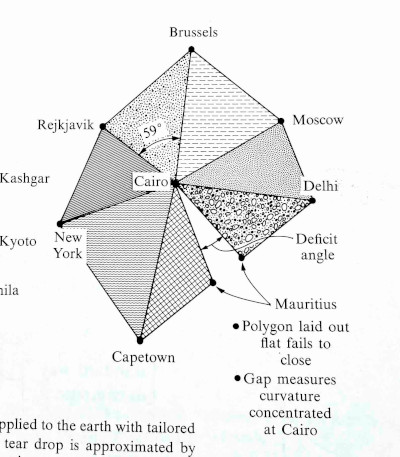The graph is centered at Cairo, Egypt and includes triangles whose side lengths are the distances between cities. The triangles are calculated using only distances, not by measuring angles per se.

The geometry of each triangle is Euclidean: giving the three edge lengths fixes all the features of the figure, including the indicated angle. … The triangles that belong to a given vertex [i.e. Cairo], laid out on a flat surface, fail to meet.

I will reproduce the plot in Python because I’m more familiar with making plots there. But I’ll get the geographic data out of Mathematica, because I know how to do that there.

## Geographic information from Mathematica

I found the distances between the various cities using the GeoDistance function in Mathematica. The arguments to GeoDistance are “entities” which are a bit opaque. When using Mathematica interactively, you can use ctrl + = to enter the name of an entity. There’s some guesswork, e.g. whether I meant New York City or the state of New York when I entered “New York”, but Mathematica guessed correctly. The following code lists the city entities explicitly.

    cities = {
Entity["City", {"Cairo", "Cairo", "Egypt"}],
Entity["City", {"Delhi", "Delhi", "India"}],
Entity["City", {"Moscow", "Moscow", "Russia"}],
Entity["City", {"Brussels", "Brussels", "Belgium"}],
Entity["City", {"Reykjavik", "Hofudhborgarsvaedhi", "Iceland"}],
Entity["City", {"NewYork", "NewYork", "UnitedStates"}],
Entity["City", {"CapeTown", "WesternCape", "SouthAfrica"}],
Entity["City", {"PortLouis", "PortLouis", "Mauritius"}] }


Most of these are predictable, but I would not have guessed the code for Reykjavik or Cape Town. I found these by using the command InputForm and entering the entities as above.

I found the distance from Cairo to each of the other cities with

    Table[GeoDistance[cities[], cities[[i]]], {i, 2, 8}]

and the distances from the cities to their neighbors with

    Table[GeoDistance[cities[[i]], cities[[i + 1]]], {i, 2, 7}]
GeoDistance[cities[], cities[]]


## Drawing the plot

Now that we’ve got the data, how do we draw the plot?

Let’s put Cairo at the origin. First we draw a line from Cairo to Delhi. We might as well put Delhi on the x-axis to make things simple.

Next we need to plot Moscow. We know the distance R1 from Cairo to Moscow, and the distance R2 from Delhi to Moscow. So imagine drawing a circle of radius R1 centered at Cairo and a circle of radius R1 centered at Delhi. Moscow is located where the two circles intersect. The previous post shows how to find the intersection of circles.

The two circles intersect in at two points, so which do we choose? We choose the intersection point that preserves the orientation of the original graph (and the globe). As we go through the cities in counterclockwise order, the cross product of the previous line to the next line should have positive z component.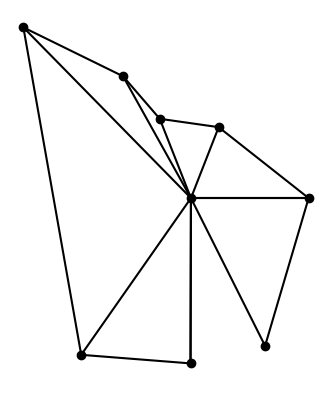This shows that the original graph was not to scale, though the gap between triangles was approximately to scale. In hindsight this should have been obvious: Brussels and Reykjavik are much closer to each other than Capetown and New York are.

## The gap

Why the gap? Because the earth is curved at Cairo (and everywhere else). If the earth were flat, the triangles would fit together without any gaps.

There’s no gap when you take spherical triangles on the globe. But even though the triangles preserve length when projected to the plane, they cannot preserve angles too. The sum of the angles in a spherical triangle adds up to more than 180°, and the amount by which the sum exceeds 180° is proportional to the size of the spherical triangle. Since the angles of triangles in the plane do add up to 180°, each flat triangle fails to capture a bit of the corresponding spherical triangles, and the failures add up to the gap we see in the image.

 Gravitation by Misner, Thorne, and Wheeler. 1973.

# Calculating the intersection of two circles

Given the equations for two circles, how can you tell whether they intersect? And if they do intersect, how do you find the point(s) of intersection?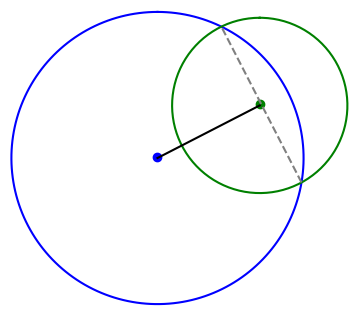MathWorld gives a derivation, but I’d like to add the derivation there in two ways. First, I’d like to be more explicit about the number of solutions. Second, I’d like to make the solution more general.

The derivation begins with the simplifying assumption that one circle is centered at the origin and the other circle is centered somewhere along the x-axis. You can always change coordinates so that this is the case, and doing so simplifies the presentation. Undoing this simplification is implicitly left as an exercise to the reader. I will go through this exercise here because I want a solution I can use in software.

## Finding the x coordinate

Suppose the first circle, the one centered at the origin, has radius R. The other circle is centered at (d, 0) for some d, and has radius r. The  x-coordinate of the intersection is shown to satisfy the following equation.When I got to this point in the derivation I was wondering what assumption was made that guaranteed there is a solution. Clearly if you increase d enough, moving the second circle to the right, the circles won’t intersect. And yet the derivation for x always succeeds, unless d = 0. If d does equal 0, the two circles are concentric. In that case they’re the same circle if R = r; otherwise they never intersect.

## Finding the y coordinate

Why does the derivation for x always succeed even though the intersection might be empty? The resolution depends on the solution for y. What we’ve found is that if the circles intersect, the x coordinate of the point(s) of intersection is given by the equation above.

The y coordinate of the point(s) of intersection satisfiesIf the numerator is negative, there is no real solution, no intersection. If the numerator is zero, there is one solution, and the two circles are tangent. if the numerator is positive, there are two solutions.

The distance between the two intersection points, if there are two intersection points, is a = 2|y|. This will be needed below.## General position

Now suppose we’re first circle is centered at (x0, y0) and the second circle is centered at (x1, y1). Again we let d be the distance between the centers of the circles. The circles intersect twice if d < R + r, once if d = R + r, and never if d > R + r.

Imagine for a moment shifting and rotating the plane so that (x0, y0) goes to the origin and goes to (d, 0). The length of the line segment between the two intersection points is still given by a above. And the distance from the center of the first circle to that line segment is given by the equation for x above.

So to find the points of intersection, we first form a unit vector in the direction of the center of the first circle headed toward the center of the second circle. This is the black line at the top of the post. We move a distance x along this line, with x as in the equation above, and then move perpendicularly a distance a/2 in either direction. This is the dashed gray line.

## Python code

The following code implements the algorithm described above.

    def circle_intersect(x0, y0, r0, x1, y1, r1):
c0 = np.array([x0, y0])
c1 = np.array([x1, y1])
v = c1 - c0
d = np.linalg.norm(v)

if d > r0 + r1 or d == 0:
return None

u = v/np.linalg.norm(v)
xvec = c0 + (d**2 - r1**2 + r0**2)*u/(2*d)

uperp = np.array([u, -u])
a = ((-d+r1-r0)*(-d-r1+r0)*(-d+r1+r0)*(d+r1+r0))**0.5/d
return (xvec + a*uperp/2, xvec - a*uperp/2)


## Application

This post started out to be part of the next post, but it turned out to be big enough to make its own post. The next post looks carefully at an example that illustrates how you could discover that you’re living on a curved surface just by measuring the distances to points around you. I needed the code in this post to make the image in the next post.

# The numerical range ellipse

Let A be an n × n complex matrix. The numerical range of A is the image of x*Ax over the unit sphere. That is, the numerical range of A is the set W(A) in defined by

W(A) = {x*Ax | x ∈ ℂn and ||x|| = 1}

where x* is the conjugate transpose of the column vector x.

The product of a row vector and a column vector is a scalar, so W(A) is a subset of the complex plane ℂ.

When A is a 2 × 2 matrix, W(A) is an ellipse, and the two foci are the eigenvalues of A.

Denote the two eigenvalues by λ1 and λ2 . Denote the major and minor axes of the ellipse by a and b respectively. Then

b² = tr(A*A) − |λ1|² − |λ2|².

where tr is the trace operator. This is the elliptical range theorem . The theorem doesn’t state the major axis a explicitly, but it can be found from the two foci and the minor axis b.

## Demonstration

We will create a visualization the numerical range of a matrix directly from the definition, generating x‘s at random and plotting x*Ax. Then we draw the ellipse described in the elliptical range theorem and see that it forms the boundary of the random points.

The following code plots 500 random points in the numerical range of a matrix formed from the numbers in today’s date. The eigenvalues are plotted in black.

import numpy as np
from scipy.stats import norm
import matplotlib.pyplot as plt

A = np.array([[8, 16], [20, 23j]])

for _ in range(5000):
v = norm(0, 1).rvs(size=4)
v /= np.linalg.norm(v)
x = np.array([v + 1j*v, v + 1j*v])
z = np.conj(x).dot(A.dot(x))
plt.plot(z.real, z.imag, 'o', color='0.9')

eigenvalues, eigenvectors = np.linalg.eig(A)
plt.plot(eigenvalues.real, eigenvalues.imag, 'ko')
plt.plot(eigenvalues.real, eigenvalues.imag, 'ko')


The following code draws the ellipse guaranteed by the elliptical range theorem.

A_star_A = np.dot(np.conj(A).T, A)
b = (np.trace(A_star_A) - abs(eigenvalues)**2 - abs(eigenvalues)**2)**0.5
c = 0.5*abs(eigenvalues - eigenvalues)
a = (2/3)*(2*(2*b**2/4 + c**2)**0.5 + c)

t = np.linspace(0, np.pi*2)
z = a*np.cos(t)/2 + 1j*b*np.sin(t)/2
u = (eigenvalues - eigenvalues)/2
u /= np.linalg.norm(u)
m = eigenvalues.mean()
z = z*u + m

plt.plot(z.real, z.imag, 'b-')


Here is the result.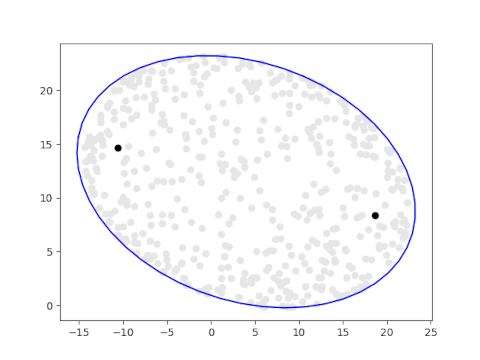Here is the result of running the same code with 10,000 random points.## Related posts

 Ulrich Daepp, Pamela Gorkin, Andrew Shaffer, and Karl Voss. Finding Ellipses: What Blaschke Products, Poncelet’s Theorem, and the Numerical Range Know about Each Other. MAA Press 2018.

# Random slices of a sphube

Ben Grimmer posted something yesterday on Twitter:

A nice mathematical puzzle🧐

If you take a 4-norm ball and cut it carefully, you will find a two-norm ball. 3D printed visual evidence below.
The puzzle: Why does this happen and how much more generally does it happen?

(This question was first posed to me by Pablo Parrilo)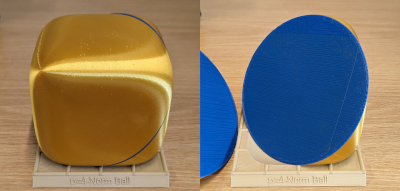This is a surprising result, and it touches on two things I’ve written about before.

Several years ago I wrote about the surprising result that if you slice open a Menger sponge a certain way then you can see six-pointed stars. I was just as surprised to see the result above. I also wrote several posts about “squircles,” and the 4-norm ball is a 3D version of the squircle, sometimes called a “sphube.”

I modified the code I’d written for the Menger sponge post to create random slides of the 4-norm ball. Here are some of the results.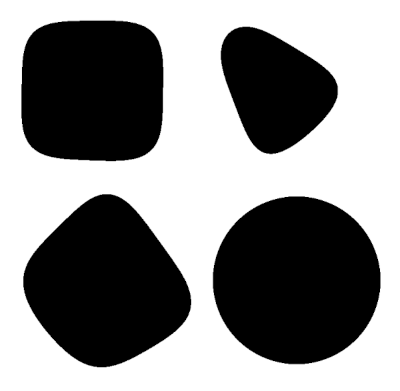One of these happens to be a circle, or at least close enough that it looks like a circle.

My code generated a random point and a random normal vector to determine a plane through that point. That’s how I produced the square-ish and triangle-ish plots.

For the last image, I set the normal vector to (1, 1, 1) because the 3D printed image above suggested that would be the right direction. I generated random points inside the cube, and one time the random point was approximately (0.2, 0, -0.2) and noticed the plot was approximately circular. It seems that when z = −x and the normal vector is (1, 1, 1) you get a circular cross section. By symmetry you can make many more examples from this example.

I have not proved anything yet; just played with plots.

# Spherical coordinate Rosetta Stone

If you’ve only seen one definition of spherical coordinates, you may be shocked to discover that there are multiple conventions. In particular, mathematicians and geoscientists have different conventions. As Volker Michel put it in book on constructive approximation,

Many mathematicians have faced weird jigsaw puzzles with misplaced continents after using a data set from a geoscientist.

Nor are there only two conventions. This post will cover three conventions, which I will call physics, math, and geography. This is not to imply that everyone in a profession uses the conventions named after the profession.

As with Fourier transforms there are many conventions. Presumably the reason for the proliferation of conventions is the same: a useful basic concept will be discovered multiple times in different areas of application. When there are trade-offs between conventions, people working in each area will choose the convention that is simplest for the things they care most about.

## Questions and definitions

Given a triple of numbers (c1, c2, c3), that represent spherical coordinates, there are two basic questions:

1. What do the numbers mean?
2. What symbol should be used to represent them?

Part of the meaning question is whether the coordinate system is left-handed or right-handed. There is also a minor question of the standard range of equivalent angles.

Polar angle is the angle down from the positive z-axis, the north pole. The north pole has polar angle 0 and the south pole has polar angle π.

Latitude is the angle up from the xy plane or the equator. The latitude of the north pole is π/2 and the latitude of the south pole is −π/2.

Azimuth is the angle from an initial meridian (e.g. the Prime Meridian in geography). In mathematical terms it is the angle between the x-axis and a line connecting the projection of a point to the xy to the origin.

## Physics convention

The physics convention is also the ISO 80000-2 convention. The three coordinates are

(radial distance to origin, polar angle, azimuthal angle)

and are conventionally denoted

(r, θ, ϕ).

The point (r, θ, ϕ) corresponds to

(r sin θ cos ϕ, r sin θ sin ϕ, r cos θ)

in Cartesian coordinates.

The coordinate system is right-handed: ϕ increases in the counterclockwise direction when looking down on the xy plane.

The polar angle typically runs from 0 to π and the azimuthal angle typically runs from 0 to 2π.

## Math convention

In this convention the three coordinates are

(radial distance to origin, azimuthal angle, polar angle)

and are denoted

(ρ, θ, ϕ).

When compared to the physics notation we have something of a Greek letter paradox. Both conventions agree that the second coordinate should be written θ and the third coordinate should be written ϕ, but the two conventions mean opposite things by the two symbols.

The advantage of using θ to denote the azimuthal angle is that then θ has the same meaning as it has in polar coordinates. Similarly, the advantage of using ρ rather than r is to preserve the meaning of r in polar and cylindrical coordinates: ρ is the distance in 3 dimensions from a point to the origin, and r is the distance in 2 dimensions from the projection of a point to the xy plane and the origin.

Sometimes you’ll see r rather than ρ for the first coordinate.

The point (ρ, θ, ϕ) corresponds to

(ρ cos θ sin ϕ, ρ sin θ sin ϕ, ρ cos ϕ).

The math convention uses the same orientation and typical range coordinate ranges as the physics convention.

## Geography and geoscience

Geographic coordinate systems are complicated by the fact that the earth is not perfectly spherical. Geographic latitude and geocentric latitude are not exactly the same as the following highly exaggerated diagram shows.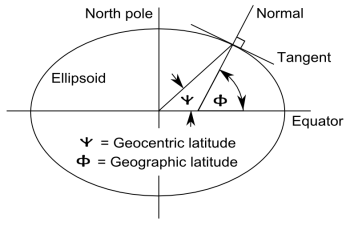However, for this post we will assume a perfectly spherical earth in which geographic and geocentric latitudes are the same.

A point on the earth’s surface is described by two numbers, latitude and longitude. This corresponds to spherical coordinates in which the first coordinate is implicitly the earth’s radius. You’ll see latitude and longitude listed in either order.

The polar angle is sometimes called colatitude because latitude is the complementary angle of latitude. Longitude corresponds to azimuth. There are multiple symbols used for latitude, though λ is common for longitude. If you see one coordinate denoted λ, the other is latitude.

The safest, most explicit notation is to use the words longitude and latitude, not depending on symbols or order. Longitude is implicitly longitude east of the Prime Meridian, but to be absolutely clear, put an E at the end. Latitude typically ranges from −π/2 to π/2 (−90° to 90°). Longitude either runs from 0 to 2π or from −π to π (−180° to 180°).

To convert to Cartesian coordinates, set polar angle to π/2 − latitude, azimuth to longitude (east), and use the formulas above for either the physics or mathematics notation. If you have elevation, this is the radial distance above the earth’s surface, so ρ is the earth’s radius plus elevation.

# Area and volume of hypersphere cap

A spherical cap is the portion of a sphere above some horizontal plane. For example, the polar ice cap of the earth is the region above some latitude. I mentioned in this post that the area above a latitude φ iswhere R is the earth’s radius. Latitude is the angle up from the equator. If we use the angle θ down from the pole, we getI recently ran across a generalization of this formula to higher-dimensional spheres in . This paper uses the polar angle θ rather than latitude φ. Throughout this post we assume 0 ≤ θ ≤ π/2.

The paper also includes a formula for the volume of a hypersphere cap which I will include here.

## Definitions

Let S be the surface of a ball in n-dimensional space and let An(R) be its surface area.Let Ix(a, b) be the incomplete beta function with parameters a and b evaluated at x. (This notation is arcane but standard.)This is, aside from a normalizing constant, the CDF function of a beta(a, b) random variable. To make it into the CDF, divide by B(a, b), the (complete) beta function.## Area equation

Now we can state the equation for the area of a spherical cap of a hypersphere in n dimensions.Recall that we assume the polar angle θ satisfies 0 ≤ θ ≤ π/2.

It’s not obvious that this reduces to the equation at the top of the post when n = 3, but it does.

## Volume equation

The equation for the volume of the spherical cap is very similar:where Vn(R) is the volume of a ball of radius R in n dimensions.## Related posts

 Shengqiao Li. Concise Formulas for the Area and Volume of a Hyperspherical Cap. Asian Journal of Mathematics and Statistics 4 (1): 66–70, 2011.

# Random points in a high-dimensional orthant

In high dimensions, randomly chosen vectors are very likely nearly orthogonal. I’ll unpack this a little bit then demonstrate it by simulation. Then I’ll look at what happens when we restrict our attention to points with positive coordinates.

***

The lengths of vectors don’t contribute to the angles between them, so we may as well focus our attention on spheres.

Suppose we pick two points on a familiar sphere in 3 dimensions, our home planet. By symmetry, we can assume the first vector runs from the center of the earth to the north pole. Otherwise we can imagine picking the first point, then rotating the earth so that the chosen point becomes the north pole.

What vectors are orthogonal (perpendicular) to the north pole? The vectors from the center to a point on the equator. The vectors that are nearly orthogonal to the north pole are the vectors that are near the equator.

On a high-dimensional sphere, nearly all the area is near the equator (more on that here). This means that if we choose two vectors, they are very likely to be nearly orthogonal.

## Simulating points on sphere

I’ll illustrate this by choosing pairs of points at random on a unit sphere in 100 dimensions and computing their dot product. This gives the cosine similarity of the points, the cosine of the angle between them. I’ll repeat this process 1,000 times and look at the average cosine similarity.

    from scipy.stats import norm
import numpy as np

np.random.seed(20230809)

numruns = 1000
avg = 0
dim = 100
for _ in range(numruns):
x = norm(0,1).rvs(size = dim)
y = norm(0,1).rvs(size = dim)
avg += np.dot(x, y)/(np.dot(x,x)*np.dot(y,y))**0.5
print(avg/numruns)


Here we use the standard method of generating random points on a sphere: generate each component as a normal (Gaussian) random variable, then divide by the length. The code above combines the normalization with finding the dot product.

The code above prints 0.00043. The average dot product between vectors is very small, i.e. the vectors are nearly orthogonal.

## Simulating points in orthant

Next let’s look at one orthant of the sphere. (“Orthant” is the generalization to higher dimensions of quadrant in two dimensions and octant in three dimensions.) We’ll generate random samples from the first orthant by first generating random samples from the whole sphere, then taking the absolute value to fold all the points from the other orthants into the first orthant.

The only necessary change to the code is to put abs() around the code generating the random points.

    x = abs(norm(0,1).rvs(size = dim))
y = abs(norm(0,1).rvs(size = dim))


When we run the code again it prints 0.639. This is the average value of the cosine of the angle between points, so the average angle is about 0.877 radians or about 50°.

This isn’t quite the right thing to do. We should convert to angles first, then take the average. But that leads to essentially the same result.

If my pencil-and-paper calculations are correct, the exact expected dot product value approaches 2/π = 0.6366 as dimension goes to infinity, and the value of 0.639 above would be consistent with this.